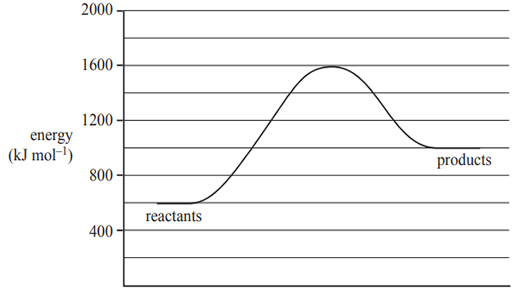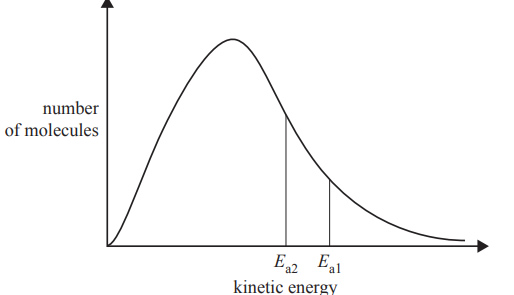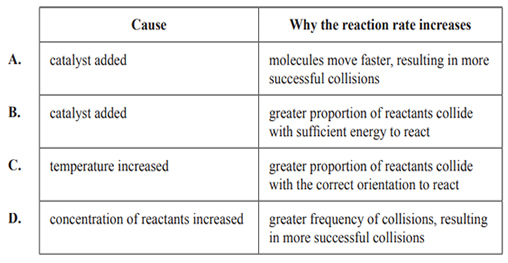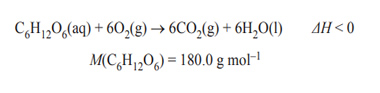Thermochemistry (2018 NHT) 1) The following diagram shows the energy profi le for a reaction.A catalyst reduces the activation energy by 250 kJ mol–1. The value of the enthalpy change, in kJ mol–1, of the catalysed reaction is A. –600 B. 400 C. 750 D. 1000 Solution2) The diagram below represents the distribution of kinetic energy in a sample of gaseous reactant molecules. Activation energy Ea1 can be changed to activation energy Ea2.This change increases the reaction rate. Which of the following gives the most likely cause of the change from Ea1 to Ea2 and explains why the reaction rate would increase?Solution3) The equation for cellular respiration is as follows.When 72.0 g of glucose, C6H12O6, is metabolised in cellular respiration, the total energy released is 1.126 × 103 kJ. The value of ΔH, in kJ mol–1, for the equation above is A. –1.56 × 101 B. –4.50 × 102 C. –2.82 × 103 D. –8.11 × 104 Solution